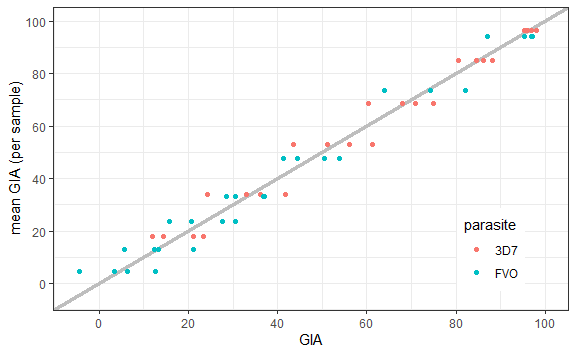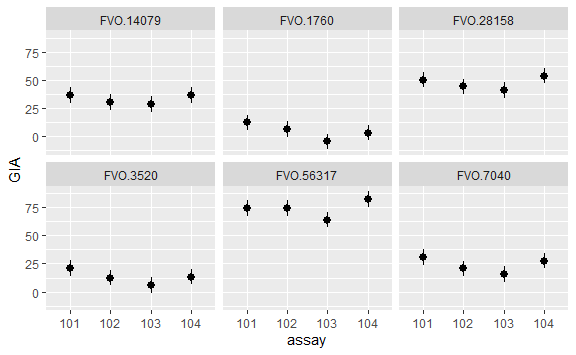# Validation of Growth Inhibition Assay using testassay

## Introduction

The growth inhibition assay (GIA) is a functional assay that measures how antibodies (immunoglobulin G, IgG) in a blood sample inhibits the growth (and/or invasion) of certain malaria parasites. It is a functional assay in the sense that it is designed to measure the function of a sample, rather than the amount of a specifc analyte. The growth inhibition assay is described in detail in (Malkin et al. 2005). Briefy, the purified IgG from the test sample is mixed with malaria-infected red blood cells (RBCs) in a well of a 96 well plate. A negative control is a well with infected RBCs without test IgG on the same plate.

The amount of parasite growth in either of those wells is measured by a biochemical assay specific for parasite lactate dehydrogenase using optical density wavelength of 650 (OD650). Specifically, the GIA from those two wells after adjusting for the OD650 from normal RBCs is

$GIA = 100 \left( 1 - \frac{OD_{650} \mbox{ of infected RBCs with test IgG } - OD_{650} \mbox{ of normal RBCs} }{OD_{650} \mbox{ of infected RBCs without any IgG } - OD_{650} \mbox{ of normal RBCs} } \right).$

Following (Cummings et al. 2010) we want to focus our validation process on the intended purposes of the GIA. One main purpose for the assay is to determine whether a given sample has any growth inhibition, and if so, how much. So the general purpose standard deviation interval should be useful in showing the middle 68.27% probable range of any sample. So the effective standard deviation of $$Y$$ will be a useful statistic.

## Analysis

For this demonstration, we use GIA replicate measurements on samples, where the GIA is based on two different strains of the Plasmodium falciparum parasite, 3D7 and FVO. Each sample is measured 4 times on 4 different assays. There are 6 samples measured using the 3D7 strain, and in each of the 4 assays each of the 6 samples is measured once. Similarly, there are 7 samples measured using the FVO strain, and in each of 4 different assays each of the 7 samples is measured once. There is statistical dependence due to the samples being all measured on the same assay, so in a proper qualifying procedure each replicate would be measured on a different assay. For the purposes of illustration assume that each replicate is measured on a different assay.

The example data are included with the package in the object called gia. This contains the raw elisa and gia values, for each replicate along with the sample-level means and variances.

summary(gia)
##  parasite     assay        elisa             gia           sample
##  3D7:24   101    : 7   Min.   :  1760   Min.   :-4.36   Length:52
##  FVO:28   102    : 7   1st Qu.:  4098   1st Qu.:22.91   Class :character
##           103    : 7   Median : 14079   Median :44.08   Mode  :character
##           104    : 7   Mean   : 27123   Mean   :49.58
##           1      : 6   3rd Qu.: 32786   3rd Qu.:76.31
##           2      : 6   Max.   :112633   Max.   :97.92
##           (Other):12
##    meanAAgia         varAAgia
##  Min.   : 4.505   Min.   : 1.28
##  1st Qu.:23.655   1st Qu.:22.43
##  Median :47.535   Median :38.14
##  Mean   :49.580   Mean   :34.96
##  3rd Qu.:73.672   3rd Qu.:49.29
##  Max.   :96.585   Max.   :56.50
## 

In the following figure we plot the mean of the 4 replicates for each sample by its 4 GIA measurements. The constant standard deviation model on the GIA appears reasonable except for very large values of mean GIA (above about 80% the variance looks smaller). Thus, we consider only the range with mean GIA $$<80\%$$. For these examples, we use the $$m\!:\!n\!:\!90\%$$ procedures, but the $$m\!:\!n\!:\!80\%$$ ones could have also been used.We start with the 3D7 GIA assays. The range with mean GIA $$<80\%$$ leaves us with $$m=4$$ levels for testing. We run a $$4\!:\!4\!:\!90\%$$ procedure with a constant normal variance model. Using the testassay function from the synonymous package, we pass the parameters $$m$$, $$n$$, and $$q$$ as arguments. We also must specify the model type as either “normal”, “lognormal”, or “gamma”, and whether the variance is constant or the CV is constant.

treD7.test <- testassay(x = gia,
m = sample, n = assay, q = .9,
model = "normal", constant = "variance",
data = subset(gia, parasite == "3D7" & meanAAgia < 80))

treD7.test
## Results of a 4:4:90 % procedure.
## Assuming a normal model with constant SD
##
##      sample    mean       sd     U.SD
## 1  3D7.2049 17.7525 5.477849 5.758026
## 2  3D7.4098 33.8225 7.354814 7.730994
## 3  3D7.8196 53.0775 7.516841 7.901308
## 4 3D7.16393 68.4850 6.176150 6.492045
##
##  U.SD is the one-sided upper confidence limit at alpha = 0.4376587
## Between assay values of 17.7525 to 68.485 the assay passes the procedure with bound 7.901308
## Use predict to obtain confidence intervals for future observations

so we calculate confidence intervals for $$\sigma$$ at the one-sided $$1 - (.10)^{1/4}$$ = 0.4376587 level. These are given as U.sd in the table printed out by testassay.

Although, 0.4376587 seems like a strange level for the individual upper limits, it allows us to take the maximum as a 90% limit, so that $$\bar{\sigma}(.90)$$ = 7.9013076. Although the effective standard deviation was calculated without using the values with mean GIA $$>80\%$$, since the variance of those values appears less, we can practically extend the range of the precision up to $$100\%$$.

We can use the predict method to report observed assay values as confidence intervals with the effective standard deviation. We simply pass a vector of observations to the predict function on the assaytest object. If newdata is not given, it will report the intervals for the values used in the validation procedure. Be aware that the predict procedure is not aware of any upper or lower limits to the assay, so interpret the results with care. For instance, the GIA assay has an upper limit of 100, but the software does not restrict the confidence interval upper limits so they could potentially be above 100.

obsD7 <- rnorm(5, mean = 50, sd = 18)
predict(treD7.test, newdata = obsD7)
## Warning in predict.assaytest(treD7.test, newdata = obsD7): New observations
## outside the range of the assay validation procedure!
##        obs     lower    upper
## 1 57.31050 49.409193 65.21181
## 2 71.94920 64.047897 79.85051
## 3 72.99197 65.090663 80.89328
## 4 38.19940 30.298089 46.10070
## 5 15.66942  7.768116 23.57073

Now we run the procedure for the FVO samples.

FVO.test <- testassay(x = gia,
m = sample, n = assay, q = .9,
model = "normal", constant = "variance",
data = subset(gia, parasite == "FVO" & meanAAgia < 80))

FVO.test
## Results of a 6:4:90 % procedure.
## Assuming a normal model with constant SD
##
##      sample    mean       sd     U.SD
## 1  FVO.1760  4.5050 7.020795 6.485295
## 2  FVO.3520 13.1325 6.326929 5.844351
## 3  FVO.7040 23.6550 6.719670 6.207137
## 4 FVO.14079 33.2600 4.290322 3.963084
## 5 FVO.28158 47.5350 5.734280 5.296906
## 6 FVO.56317 73.6725 7.468335 6.898699
##
##  U.SD is the one-sided upper confidence limit at alpha = 0.3187079
## Between assay values of 4.505 to 73.6725 the assay passes the procedure with bound 6.898699
## Use predict to obtain confidence intervals for future observations
predict(FVO.test)
## Warning in predict.assaytest(FVO.test): New observations outside the range of
## the assay validation procedure!
##      obs       lower     upper
## 1  74.27  67.3713013 81.168699
## 2  50.52  43.6213013 57.418699
## 3  36.84  29.9413013 43.738699
## 4  30.64  23.7413013 37.538699
## 5  21.13  14.2313013 28.028699
## 6  12.54   5.6413013 19.438699
## 7  74.31  67.4113013 81.208699
## 8  44.52  37.6213013 51.418699
## 9  30.59  23.6913013 37.488699
## 10 20.72  13.8213013 27.618699
## 11 12.39   5.4913013 19.288699
## 12  6.40  -0.4986987 13.298699
## 13 63.95  57.0513013 70.848699
## 14 41.20  34.3013013 48.098699
## 15 28.63  21.7313013 35.528699
## 16 15.72   8.8213013 22.618699
## 17  5.68  -1.2186987 12.578699
## 18 -4.36 -11.2586987  2.538699
## 19 82.16  75.2613013 89.058699
## 20 53.90  47.0013013 60.798699
## 21 36.98  30.0813013 43.878699
## 22 27.54  20.6413013 34.438699
## 23 13.33   6.4313013 20.228699
## 24  3.44  -3.4586987 10.338699

A plot of the observed values with effective standard deviation intervals:

predat <- cbind(subset(gia, parasite == "FVO" & meanAAgia < 80), predict(FVO.test))
## Warning in predict.assaytest(FVO.test): New observations outside the range of
## the assay validation procedure!
ggplot(predat,
aes(x = assay, y = obs, ymin = lower, ymax = upper)) +
geom_pointrange() + facet_wrap(~ sample) + ylab("GIA")We can also run validation procedures assuming a lognormal model, and/or constant coefficient of variation (CV), which is the standard deviation divided by the mean. A constant coefficient of variation model is appropriate when the standard deviation increases as the assay value increases. This is not the case here, but we run the model for illustration. The constant cv models give upper limits of confidence intervals for the CV instead of the SD. The effective standard deviation intervals differ depending on the model.

newobs <- c(25, 40, 65)
predict(treD7.test, newobs)
##   obs    lower    upper
## 1  25 17.09869 32.90131
## 2  40 32.09869 47.90131
## 3  65 57.09869 72.90131
cvn <- testassay(x = gia,
m = sample, n = assay, q = .9,
model = "normal", constant = "cv",
data = subset(gia, parasite == "3D7" & meanAAgia < 80))

predict(cvn, newobs)
##   obs    lower    upper
## 1  25 17.24702 36.23816
## 2  40 27.59523 57.98105
## 3  65 44.84226 94.21921
cvln <- testassay(x = gia,
m = sample, n = assay, q = .9,
model = "lognormal", constant = "cv",
data = subset(gia, parasite == "3D7" & meanAAgia < 80))

predict(cvln, newobs)
##   obs    lower    upper
## 1  25 17.31858 36.08840
## 2  40 27.70974 57.74144
## 3  65 45.02832 93.82984